## 14.7Distributed Parameter Equivalents and Models with Dissipation

The distributed parameter transmission line of Sec. 14.1 is now generalized to include certain types of dissipation by using the incremental circuit shown in Fig. 14.7.1 . The capacitance per unit length C is shunted by a conductance per unit length G and in series with the inductance per unit length L is the resistance per unit length R.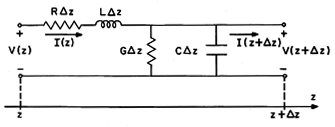Fig 14.7.1 Incremental section of lossy distributed line.

If the line really were made up of so many lumped parameter elements that it could be described by continuum equations, G would be the conductance per unit length of the lossy capacitors (Sec. 7.9) and R would be the resistance per unit length of the inductors. More often, G and R (like L and C) are either equivalent to or a model of a physical system. Examples are discussed in the next two sections.

The steps leading to the generalized transmission line equations are suggested by Eqs. 14.1.1 through 14.1.5. In requiring that the currents at the terminal on the right sum to zero, there is now an additional current through the shunt conductance. In the limit where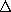z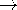0,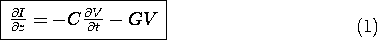Similarly, in summing the voltages around the loop, there is now a voltage drop across the series resistance. Again, in the limitz0,As should be expected, with the introduction of dissipation represented by G and R, V(z, t) and I(z, t) no longer take the form of waves propagating without distortion. That is, substitution shows that solutions no longer take the form of (14.4.1)-(14.4.3). As a result, we would have to work considerably harder than in Secs. 14.3-14.4 to describe transients on lossy transmission lines. However, although somewhat more involved then before, the sinusoidal steady state response follows from the approach illustrated in Secs. 14.5-14.6.

With the objective of describing the sinusoidal steady state, complex amplitude representations of V and I (14.5.2) are substituted into (1) and (2) to give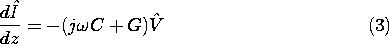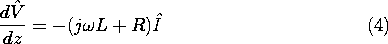To obtain an expression for the voltage alone, (3) is substituted into the derivative of (4).With the voltage found from this equation, the current follows from (4).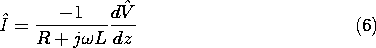Albeit complex, the coefficient in (5) is constant, so it is again appropriate to look for exponential solutions. Using the convention established in Sec. 14.5, we look for solutions exp (-jkz). Substitution into (5) then shows that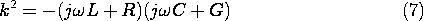So as to be clear in distinguishing the two roots of this dispersion equation, we define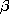as having a positive real part and write the roots as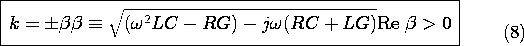These roots, k = kr + jki, are pictured as a function of frequency in Fig. 14.7.2. From (7), k2 is in the lower half-plane. It follows that the value of k having a positive real part (defined as) has a negative imaginary part.Fig 14.7.2 Roots of (7) as functions of normalized. The real and imaginary parts, respectively, of the complex wave numberrelate to the phase velocity and rate of decay of the wave, as shown.

These definitions take on physical significance when the solutions to (5) are written as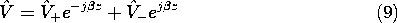because it is then clear that we have definedso that V+ represents a wave with points of constant phase propagating in the +z direction. Note that becausei is negative, this wave decays in the +z direction, as shown in Fig. 14.7.2. Similarly,- is the complex amplitude at z = 0 of a wave that decays in the -z direction and has phases propagating in the -z direction.

10 It is important not to generalize from this finding. The direction of propagation of points of constant phase (the phase propagation direction, PPG, is not, in general, indicative of the directions of propagation of the wave; i.e., a source positioned on an infinite line at z = 0 does not necessarily cause waves with positive PPG to go away from the source in the +z direction and with negative PPG to go away in the -z direction. The direction of propagation is usually determined by the direction of the group velocity (GV). In the presence of loss, waves with positive GV decay in the +z-direction, with negative GV in the -z-direction.

In terms of the two coefficients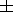, the current expression follows from substituting (9) into (6)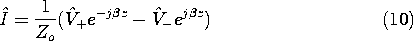where the complex characteristic impedance is now defined as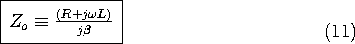Comparison of (9) and (10) with (14.5.5) and (14.5.6) shows that the impedance and reflection coefficient descriptions are applicable, provided we generalizeand Zo to be the complex numbers given by (8) and (11). That these quantities are now complex is an inconvenience

11 Circumvented by having a calculator programmed to carry out operations on complex variables. and a warning that some ideas established for the ideal line need to be reexamined. For example, reasoning as in Sec. 14.5 shows that a pure resistance can no longer be used to match the line. Further, it is not possible to match the line at all frequencies with any finite number of lumped elements.

#### Example 14.7.1. Signal Attenuation on an Open Circuit Line

The lossy transmission line shown in Fig. 14.7.3 is open at the right and driven by a voltage source of complex amplitude Vg at the left. What is the voltage measured at the open circuit?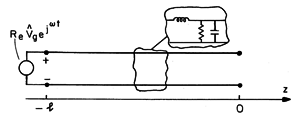Fig 14.7.3 Open circuit lossy line.

The open circuit at z = 0 requires that I(0) = 0, and hence [from (10)] that V+ = V-. Thus, the voltage, as given by (9), is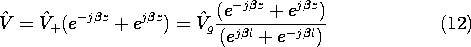Here we have adjusted the coefficient+ so that the voltage isg at the left end, where the voltage source is connected. As a function of time, the voltage distribution is therefore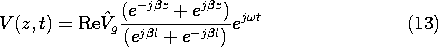Much of the complicated phenomenon represented by this simple expression is encapsulated in the complex wave number. To illustrate, consider the voltage measured at z = 0, which from (13) has the complex amplitude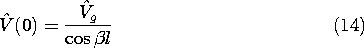If a calculator or computer is not available for evaluating the cosine of a complex number, then we can use the double-angle identity to write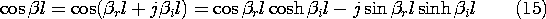For the case where all of the loss is due to the shunt conductance (R = 0), the frequency response is illustrated in Fig. 14.7.4. The four responses shown are for increasing amounts of loss, perhaps introduced by increasing G.Fig 14.7.4 Frequency response for open circuit lossy line.

At very low frequencies, the output voltage simply follows the driving voltage. This is because we have considered the case where R = 0 and with the frequency so low that the inductor has no effect, the output terminals are connected to the source through a negligible impedance.

As the frequency is raised, consider first the response with no loss (l/l* = 0). As the frequency approaches that required to make the line a quarter-wavelength long, the impedance of the line at the generator approaches zero and the current approaches infinity. This resonance condition results in the infinite response at z = 0. (As the frequency is raised further, these resonance conditions occur each time the frequency is such that the line has a length equal to a quarter-wavelength plus a multiple of a half-wavelength.) With the addition of a slight amount of loss (l/l* = 0.1), the response is finite even under the resonance conditions. Further increasing the loss (l/l* = 1) results in a response with a dull peak at the resonance point. Still larger losses (l/l* = 10) bring in skin effect and monotonic attenuation of the voltage over the length of the line. Phenomena underlying this response are discussed in the next section.